Documentation

## Modal Analysis of Identified Models

Identify state-space models of systems. Use the models to compute frequency-response functions and modal parameters. This example requires a System Identification Toolbox™ license.

### Hammer Excitation

Load a file containing three-input/three-output hammer excitation data sampled at 4 kHz. Use the first ${10}^{4}$ samples for estimation and samples $2×{10}^{4}\text{\hspace{0.17em}}$ to $5×{10}^{4}$ for model quality validation. Specify the sample time as the inverse of the sample rate. Store the data as `@iddata` objects.

```load modaldata XhammerMISO1 YhammerMISO1 fs rest = 1:1e4; rval = 2e4:5e4; Ts = 1/fs; Estimation = iddata(YhammerMISO1(rest,:),XhammerMISO1(rest,:),Ts); Validation = iddata(YhammerMISO1(rval,:),XhammerMISO1(rval,:),Ts,'Tstart',rval(1)*Ts);```

Plot the estimation data and the validation data.

```plot(Estimation,Validation) legend(gca,'show')```Use the `ssest` function to estimate a 7th-order state-space model of the system that minimizes the simulation error between the measured outputs and the model outputs. Specify that the state-space model has feedthrough.

```Orders = 7; opt = ssestOptions('Focus','simulation'); sys = ssest(Estimation,Orders,'Feedthrough',true,'Ts',Ts,opt);```

(To find the model order that gives the best tradeoff between accuracy and complexity, set `Orders` to `1:15` in the previous code. `ssest` outputs a log plot of singular values that lets you specify the order interactively. The function also recommends a model order of 7.)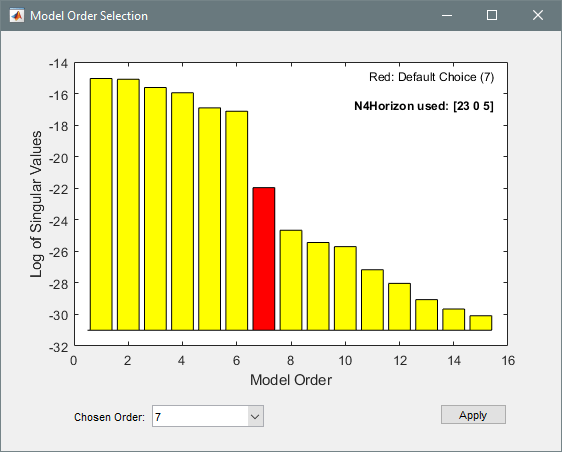Validate the model quality on the validation dataset. Plot the normalized root mean square error (NRMSE) measure of goodness-of-fit. The model describes accurately the output signals of the validation data.

`compare(Validation,sys)`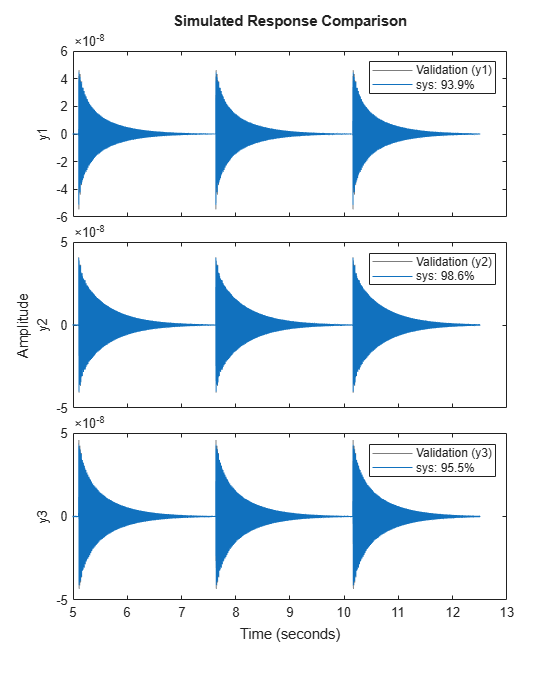Estimate the frequency-response functions of the model. Display the functions using `modalfrf` without output arguments.

```[frf,f] = modalfrf(sys); modalfrf(sys)```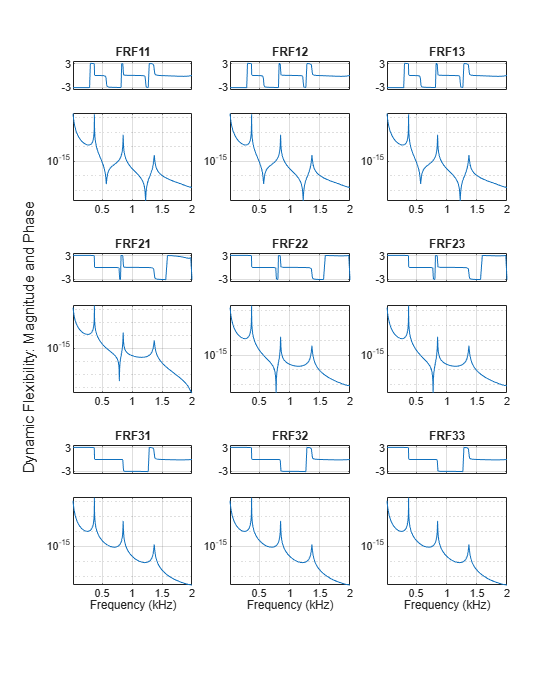Assume that the system is well described using three modes. Compute the natural frequencies, damping ratios, and mode-shape vectors of the three modes.

```Modes = 3; [fn,dr,ms] = modalfit(sys,f,Modes)```
```fn = 3×1 103 × 0.3727 0.8525 1.3706 ```
```dr = 3×1 0.0008 0.0018 0.0029 ```
```ms = 3×3 complex 0.0036 - 0.0019i 0.0039 - 0.0005i 0.0021 + 0.0006i 0.0043 - 0.0023i 0.0010 - 0.0001i -0.0033 - 0.0010i 0.0040 - 0.0021i -0.0031 + 0.0004i 0.0011 + 0.0003i ```

Compute and display the reconstructed frequency-response functions. Express the magnitudes in decibels.

```[~,~,~,ofrf] = modalfit(sys,f,Modes); clf for ij = 1:3 for ji = 1:3 subplot(3,3,3*(ij-1)+ji) plot(f/1000,20*log10(abs(ofrf(:,ji,ij)))) axis tight title(sprintf('In%d -> Out%d',ij,ji)) if ij==3 xlabel('Frequency (kHz)') end end end```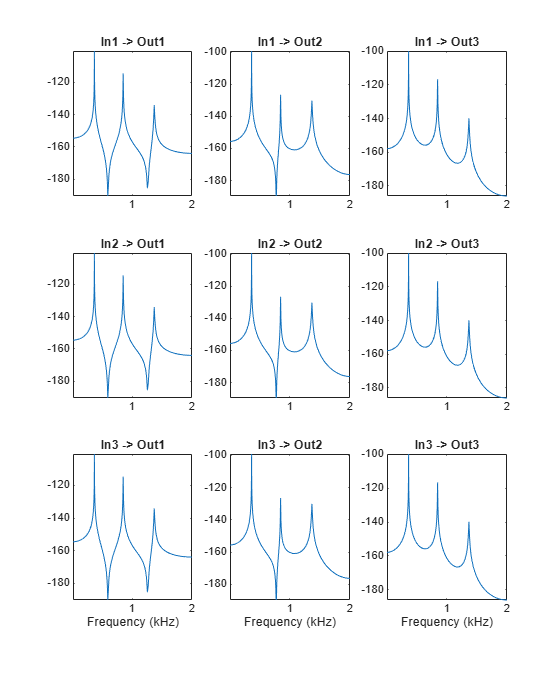### Controlled Unstable Process

Load a file containing a high modal density frequency-response measurement. The data corresponds to an unstable process maintained at equilibrium using feedback control. Store the data as an `idfrd` object for identification. Plot the Bode diagram.

```load HighModalDensData FRF f G = idfrd(permute(FRF,[2 3 1]),f,0,'FrequencyUnit','Hz'); figure bodemag(G) xlim([0.01,2e3])```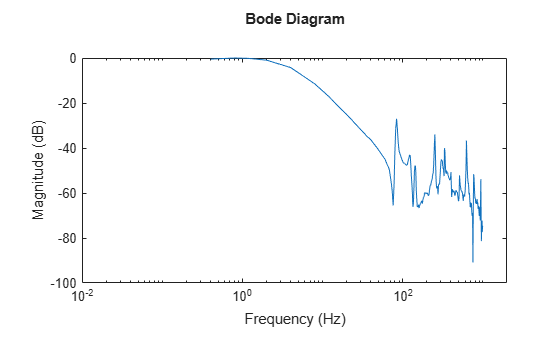Identify a transfer function with 32 poles and 32 zeros.

`sys = tfest(G,32,32);`

Compare the frequency response of the model with the measured response.

```bodemag(G,sys) xlim([0.01,2e3]) legend(gca,'show')```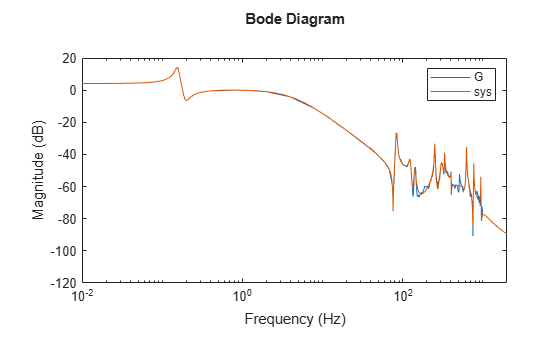Extract the natural frequencies and damping ratios of the first 10 least-damped oscillatory modes. Store the results in a table.

```[fn,dr] = modalfit(sys,[],10); T = table((1:10)',fn,dr,'VariableNames',{'Mode','Frequency','Damping'})```
```T=10×3 table Mode Frequency Damping ____ _________ _________ 1 82.764 0.011304 2 85.013 0.015632 3 124.04 0.025252 4 142.04 0.017687 5 251.46 0.0062182 6 332.79 0.0058266 7 401.21 0.0043645 8 625.14 0.0039247 9 770.49 0.002795 10 943.64 0.0019943 ```